# Travelling Sine Wave

This is a support page to the multimedia chapter Travelling Waves in the volume Waves and Sound. It gives background information and further details.

### Travelling sine wave

In the chapter on oscillations, we concentrated on sinusoidal oscillations. The reason was not only their intrinsic importance, but also that any motion can be expressed in terms of a sum of sinusoidal oscillations, using the Fourier components. Sine waves are also widespread and important. Further, any wave can be written as a sum of sine waves. Which is strong motivation to study the travelling sine wave in some detail.

First, let's write the sine wave in terms x', the coordinate moving with the wave. Concentrate on the red axes (x',t): we have a sinusoidal variation as x' varies but, in this moving frame, the curve doesn't vary with time. In this example y and x are displacement of the string and position along the string, so they are both lengths. So we can't simply write down y  =  sin x, because both the sine function and its argument are dimensionless numbers (revise Dimensions and units). So we must multiply the sine function by a constant and divide x by a constant, both constants having the dimension of length. Therefore let's write the stationary sine wave like this: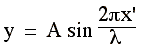.

There's a good reason for the factor of 2π. Looking at this expression, we can see that, when x' increases by λ, the argument of the sine function increases by 2π, so the sine function goes through one complete cycle. λ is called the wavelength and it can be measured, for example, as the distance between two adjacent crests.

As above, let's now write the equation in terms of the stationary coordinate x, where x'  =  x − vt :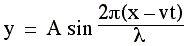.

Note that this is a function of (x − vt) and so, in the (x,y) frame, it is a wave travelling to the right with speed v.

Let's look at a point fixed on the x axis: take the origin x = 0. Substitution gives

yx=0  =  − A sin (2πvt/λ) .

We can see that this is yx=0  =  − A sin ωt, which is the equation for simple harmonic motion, with angular frequency ω  =  2πv/λ. Knowing ω we can calculate the period T  =  2π/ω  =  λ/v. Notice the negative sign: if we write the travelling sine wave as y  =  A sin (2π(x − vt)/λ), then the simple harmonic motion at the origin starts off in the negative direction. Of course, there is simple harmonic motion at all points on the travelling sine wave, with different phases from one point to the next.

We've just written T  =  2π/ω =  λ/v, which we can rearrange to give v  =  λ/T, so we have an expression for the wave speed v. In the preceding animation, we saw that, in one perdiod T of the motion, the wave advances a distance λ. This also tells us the wave speed v:

v  =  λ/T.
If we substitute for v in our equation for the travelling wave y  =  A sin (2π(x − vt)/λ, we have.

We recognise 2π/T as the angular frequency ω, defined as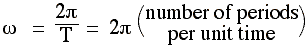.
We could think of ω as the angular frequency in time. The analogous expression in space is called the wave number, k, defined thus: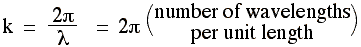.
This allows us to write the travelling sine wave in a simpler and more elegant form:
y  =  A sin (kx − ωt)
where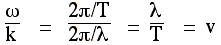, which is the wave speed.
Now let's take y  =  A sin (kx − ωt) and make the dependence on x and t explicit by plotting y(x,t) where t is a separate axis, perpendicular to x and y.

On the graph above, the purple curve, along the x axis, is a 'snapshot' of the wave at t = 0: it is the equation yt=0  =  A sin kx. Successive snapshots at later times (yt>0  =  A sin (kx − ωt)) are shown in black. The red graph, along the t axis, shows the simple harmonic motion at x = 0: it is the equation yx=0  =  − A sin ωt. Similar graphs could of course be drawn at any value of x.

### y = sin (kx − ωt)

Of course y(x,t)  =  sin (kx − ωt) is a graph in three dimensions, one of which is time. We can plot it in three spatial dimensions (or at least a 2D view of three dimensions). In the animation below, we combine the two views. At different times, we show a series of snapshots y(x) as grey curves, but we separate these along the graph's t axis. The resultant gives an impression of the three dimensional plot.

Finally, let's note that, in all of the waves shown above, we have started the clock (t = 0) when y happened to be zero. So yx=0, y=0 = 0. For the general case, we need to add a phase constant φ, just as we did when analysing simple harmonic motion. So, for the general case, we write

y  =  sin (kx − ωt + φ)
where φ is the phase constant, which can be interpreted using the initial amplitude at x = 0, i.e. yx=0,  y=0 =  A sin φ. Very often, however, we are free to choose the initial condition, so we usually choose φ = 0.

### Phases in a travelling wave: the surfer problem

 This is the problem we introduced in the tutorial: how far apart are points with a given phase difference? Here, the first pair of surfers are in phase, the second pair have a phase difference of π. Try to answer the question before going to this link for the answer.This work is licensed under a Creative Commons License.